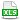HomeTechniques and Tips@RISK Simulation: Numerical ResultsStatic Value of Output Differs from Simulated Mean

# 6.4. Static Value of Output Differs from Simulated Mean

Applies to:
@RISK, all releases
@RISKOptimizer, all releases
@RISK Developer's Kit (RDK), all releases

In Simulation Settings » When a Simulation is Not Running, Distributions Return, I selected Expected Value or True EV. When a simulation is not running, the expected values of my inputs and outputs are visible in my model. I run a simulation, and the simulated means for my inputs closely match the expected values, but the simulated means of my outputs are very different from the expected values. What is wrong?

This is normal behavior.

When a simulation is not running, both inputs and outputs display their static values. For inputs, the static values are the means (unless you changed that — see Setting the "Return Value" of a Distribution). For outputs, the static values are not the means, but are values calculated from the displayed values of the inputs. In general, the mean of a calculated result does not equal the value you would calculate from the mean inputs, and the same is true for percentiles and mode.

If you want to display the means of outputs in your worksheet, use the RiskMean function. For percentiles, including the median, the 50th percentile, use RiskPtoX. Please see Placing Simulation Statistics in a Worksheet. For an example, please download the attached workbook.

In the example, column C is the inputs, and column D is the outputs created from them. For this example the computation is just a square or reciprocal, but the same principle holds for the calculations in your model. Column E is the simulated mean of each output. As expected, the simulated means are quite different from the static values displayed in column D. The principle here is "the mean of the squares is different from the square of the mean."  But the simulated means are quite close to the theoretical means I calculated for those outputs in column F.

Row 4 is a standard normal distribution (mean=0) and its square (mean=1, not 0). Row 5 is a discrete uniform with two values 1 and 9 (mean=5) and its reciprocal (mean=5/9, not 1/5). Row 6 is a continuous uniform (mean=1/2) with its square (mean=1/3, not 1/4).

If you run a simulation, you can see easily that the distributions of the outputs have different shapes from the distributions of the inputs. This will always be true, to a greater or lesser extent, for a non-linear model. Since the vast majority of models are non-linear, you should expect to see the mean values of outputs be different from the static values computed from the mean inputs.

•KB144 MeanOutputs.xlsx